https://doi.org/10.5194/amt-11-1019-2018
https://doi.org/10.5194/amt-11-1019-2018

# Global spectroscopic survey of cloud thermodynamic phase at high spatial resolution, 2005–2015

David R. Thompson, Brian H. Kahn, Robert O. Green, Steve A. Chien, Elizabeth M. Middleton, and Daniel Q. Tran
Abstract

The distribution of ice, liquid, and mixed phase clouds is important for Earth's planetary radiation budget, impacting cloud optical properties, evolution, and solar reflectivity. Most remote orbital thermodynamic phase measurements observe kilometer scales and are insensitive to mixed phases. This under-constrains important processes with outsize radiative forcing impact, such as spatial partitioning in mixed phase clouds. To date, the fine spatial structure of cloud phase has not been measured at global scales. Imaging spectroscopy of reflected solar energy from 1.4 to 1.8 µm can address this gap: it directly measures ice and water absorption, a robust indicator of cloud top thermodynamic phase, with spatial resolution of tens to hundreds of meters. We report the first such global high spatial resolution survey based on data from 2005 to 2015 acquired by the Hyperion imaging spectrometer onboard NASA's Earth Observer 1 (EO-1) spacecraft. Seasonal and latitudinal distributions corroborate observations by the Atmospheric Infrared Sounder (AIRS). For extratropical cloud systems, just 25 % of variance observed at GCM grid scales of 100 km was related to irreducible measurement error, while 75 % was explained by spatial correlations possible at finer resolutions.

Share
Dates
1 Introduction

The distribution of ice, liquid, and mixed phase clouds is important for Earth's climate and planetary radiation budget . Cloud thermodynamic phase affects radiative forcing by modulating absorption of incoming solar radiation, particle evolution, and lifetime . Previous satellite observational studies have shown that clouds are shifting poleward in the northern and southern hemispheric extratropical storm tracks . Within these shifting storm tracks, climate model experiments with forcing from increased CO2 have shown losses of cloud ice phase and gains of cloud liquid phase . This makes cloud phase an important property for accurate and continuous monitoring. Moreover, recent studies indicate that spatial partitioning of ice and liquid particles within clouds has outsized influence on climate, affecting global climate model (GCM) predictions of future warming by over 1 C . However, current observing systems cannot reduce this uncertainty; they are unable to resolve differences at critical sub-100 m scales or are insensitive to mixed phase clouds altogether.

Imaging reflectance spectroscopy from 1.4 to 1.8 µm could address this gap. Prior work used this spectral interval to measure cloud phase based on the optical absorption properties of liquid and ice . The fraction of the total path absorption due to liquid (the liquid thickness fraction, or LTF) had high sensitivity to pure and mixed phases . Liquid and ice show highly diagnostic absorption shapes which are robust to potential confounding effects such as surface reflectance, observation geometry, and mismatch in particle modeling assumptions. Remote imaging spectrometers measure these spectra from cloud tops at millions of spatial locations, resolving cloud phase at scales of tens of meters . This could constrain characteristic spatial lengths of these processes. However, global spectroscopic datasets have not yet been analyzed in this way.

Here we report a global spectroscopic survey of cloud phase from 2005 to 2015 based on data from the Hyperion imaging spectrometer instrument onboard NASA's Earth Observer 1 (EO-1) spacecraft. Hyperion also provides two novel contributions beyond prior records. First, its full spectrum fitting discriminates mixed phases by directly measuring the relative contributions of physical cloud top liquid and ice absorption. Second, Hyperion measures phase at horizontal scales of 30 m. These properties allow the first rigorous characterization of the spatial scales governing cloud top thermodynamic phase. The article first describes our estimation method, and reports seasonal and latitudinal changes of cloud thermodynamic phase. We then compare these distributions to measurements by NASA's Atmospheric Infrared Sounder (AIRS) . Finally, we show the spatial scaling properties of both extratropical and tropical cloud populations. The study lays the groundwork for future orbital imaging spectrometers that can monitor cloud characteristics when cloud cover precludes their primary mission. Imaging spectroscopy investigations typically treat clouds as contamination, when in fact cloudy data can be exploited to dramatically increase these instruments' useful data yield.

2 Cloud phase estimation

## 2.1 Method

The Hyperion imaging spectrometer operated on the sun synchronous EO-1 spacecraft for over a decade prior to decommissioning in 2017. Hyperion measured reflected solar energy from approximately 400 to 2450 nm with approximately 10 nm spectral sampling. It performed targeted acquisitions for specific regions of interest, with occasional pointing off nadir. Most targets were on land, with a high concentration in the midlatitude Northern Hemisphere. There was sparser coverage of extreme latitudes and oceans, but several island targets offered a view into cloud systems over ocean (Fig. 1). Many targets of interest were revisited multiple times during the mission. Each targeted acquisition had a ground sampling distance of approximately 30 m over a cross-track width of approximately 7.5 km, and a typical along-track distance of approximately 120 km. The Jet Propulsion Laboratory (JPL) Hyperion archive included most normal science acquisitions from 2005 through 2015, with over 4.8×104 scenes and 3.7×1010 distinct spectra.

The archive was stored as standard calibrated unorthorectified data. We first applied the cloud phase retrieval algorithm of , validated in the prior work by coincident remote and in situ aircraft measurements. We defined the TOA reflectance, ρ:

$\begin{array}{}\text{(1)}& \mathit{\rho }\left(\mathit{\lambda }\right)=\mathit{\pi }L\left(\mathit{\lambda }\right)/\left(F\left(\mathit{\lambda }\right)\mathrm{cos}\left({\mathit{\theta }}_{\mathrm{0}}\right)\right),\end{array}$

where λ was the wavelength, L was the wavelength-dependent radiance at sensor, F was the extraterrestrial solar irradiance, and θ0 was the solar zenith angle. Over short intervals we modeled ρ by a linear continuum with an offset a and slope b, attenuated by one or more Beer–Lambert absorbers j. Each absorber had a bulk absorption coefficient kj and a nonnegative thickness uj:

$\begin{array}{}\text{(2)}& \mathit{\rho }\left(\mathit{\lambda }\right)=\left(a+b\mathit{\lambda }\right)\mathrm{exp}\left[-\sum _{j}{k}_{j}\left(\mathit{\lambda }\right){u}_{j}\right]\phantom{\rule{1em}{0ex}}\mathrm{for}\phantom{\rule{1em}{0ex}}{u}_{j}\ge \mathrm{0}.\end{array}$

We modeled three absorbers: atmospheric water vapor (j=1), liquid water (j=2), and ice (j=3). As in we applied a logarithmic transformation resulting in a nonnegative least-squares problem :

$\begin{array}{ll}& -\mathrm{log}\left(\mathit{\rho }\left(\mathit{\lambda }\right)\right)\approx l+m\mathit{\lambda }+\sum _{j}{k}_{j}\left(\mathit{\lambda }\right){u}_{j}\\ \text{(3)}& & \phantom{\rule{1em}{0ex}}\mathrm{for}\phantom{\rule{1em}{0ex}}l\ge \mathrm{0},\phantom{\rule{1em}{0ex}}{u}_{j}\ge \mathrm{0}\phantom{\rule{0.25em}{0ex}}\phantom{\rule{0.25em}{0ex}}\forall \phantom{\rule{0.25em}{0ex}}j.\end{array}$

Here m permitted a log-linear continuum. We represented this to the solver as two nonnegative coefficients for upward and downward slopes, one of which would evaluate to zero. The nonnegative least-squares solution provided stable global solutions without sensitivity to initialization.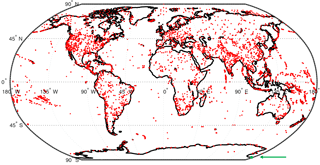Figure 1The JPL Hyperion archive comprised over 45 000 scenes, represented here by red dots. The majority were over land. A green arrow indicates the Ross Island acquisition of Fig. 2.

We calculated the H2O vapor absorption coefficients using the HITRAN 2012 line list via the Oxford Reference Forward Model (Dudhia2014). We initialized the vapor abundance using a band ratio retrieval as in and used this to calculate an “effective” absorption coefficient of band-aggregated vapor lines for use in the least-squares retrieval. Other atmospheric gases did not significantly impact the shape of vapor absorption features. We calculated liquid and ice absorption coefficients using the complex index of refraction measured by . These bulk absorption spectra were molecular properties of H2O, independent of particle size and scattering – a common practice for shortwave infrared observations of clouds . Combined with a free continuum, they fit the observed spectra of opaque clouds over short spectral intervals without precise knowledge of particle properties .

In summary, following Eq. (3) we modeled the entire interval from 1.4 to 1.8 µm with five free parameters: a continuum offset l; a slope, represented by a single degree of freedom in the variables m and n; and the vapor, ice, and liquid thicknesses uj. These thicknesses represented the length of the optical path through an equivalent homogeneous volume, as in the equivalent water thickness . As in previous work, we wrote the absorption path length u2 as the equivalent water thickness due to liquid in millimeters, EWTliquid. Similarly, u3 was the equivalent water thickness due to ice, EWTice. We then defined the liquid thickness fraction as

$\begin{array}{}\text{(4)}& \mathrm{LTF}=\frac{{\mathrm{EWT}}_{\mathrm{liquid}}}{{\mathrm{EWT}}_{\mathrm{liquid}}+{\mathrm{EWT}}_{\mathrm{ice}}}.\end{array}$

Prior in situ validation had demonstrated a robust relationship between the LTF and thermodynamic phase . We emphasize that “thickness” referred to the absorption along the optical path; clouds were heterogeneous, so the LTF was not necessarily related to their vertical dimension. In opaque clouds the measurement would be most sensitive to the upper layers.

We calculated the LTF of locations flagged by the Hyperion cloud detection algorithm of . In the Griffin et al. approach, a decision tree of threshold tests sorted spectra into different cloud types (including low and high clouds) as well as different land cover types (including snow, open terrain surfaces, and vegetation). This favored opaque clouds and generally ignored ambiguous translucent clouds that would be difficult to distinguish from land. The Griffin et al. algorithm defined tests using the top-of-atmosphere (TOA) reflectance ρ and three intermediate quantities, the normalized difference snow index (NDSI), the desert sand index (DSI), and the vegetation index (VI):

$\begin{array}{}\text{(5)}& & \mathrm{NDSI}=\left[\mathit{\rho }\left(\mathrm{0.55}\right)-\mathit{\rho }\left(\mathrm{1.65}\right)\right]/\left[\mathit{\rho }\left(\mathrm{0.55}\right)+\mathit{\rho }\left(\mathrm{1.65}\right)\right],\text{(6)}& & \mathrm{DSI}=\left[\mathit{\rho }\left(\mathrm{0.86}\right)-\mathit{\rho }\left(\mathrm{1.65}\right)\right]/\left[\mathit{\rho }\left(\mathrm{0.86}\right)+\mathit{\rho }\left(\mathrm{1.65}\right)\right],\text{(7)}& & \mathrm{VI}=\mathit{\rho }\left(\mathrm{0.66}\right)/\mathit{\rho }\left(\mathrm{0.86}\right).\end{array}$

The method was originally formulated as a flowchart but was tantamount to an ordered sequence of tests (Table 1). It evaluated each spectrum independently, applying appropriate thresholds depending on whether the scene was over ocean or land. It ascribed a classification based on the first test that evaluated to true. Random manual validation of selected scenes demonstrated adequate performance, outside the brightest glacial scenes in Antarctica.

After calculating LTF maps over all cloud areas, we aggregated counts of liquid and ice clouds. LTF was a continuous-valued quantity so the maps revealed both pure and mixed phases. We binned LTF values in 10 % graduations but also calculated binary classification using a 50 % threshold. This facilitated comparisons with historical datasets of hard categorical classifications. We then analyzed zonal distributions, estimating confidence intervals with nonparametric bootstrap variance estimation that resampled the dataset 10 000 times with replacement.

Table 1Hyperion cloud detection algorithm . We test each criterion in sequence, starting from the top, and ascribe a classification based on the first test that evaluates to true.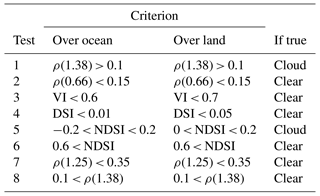Evaluating the model fit to the spectrum demanded special care, since the global catalogue included many observing geometries, terrain surface types, and potential variability in instrument calibration over the decadal record. To account for this, we estimated the noise level independently for each integration timestep and each spectral channel. We used the common method of pairwise differences between spectra at neighboring locations . Since the spatial field was mostly uniform over small distances, these differences conservatively estimated the measurement noise σ in each channel. For n cross-track locations, we applied the nonparametric variance estimate of , reprised in :

$\begin{array}{}\text{(8)}& {\stackrel{\mathrm{^}}{\mathit{\sigma }}}^{\mathrm{2}}=\frac{\mathrm{1}}{\mathrm{2}\left(n-\mathrm{1}\right)}\sum _{i=\mathrm{1}}^{n-\mathrm{1}}{\left({\mathit{\rho }}_{i+\mathrm{1}}-{\mathit{\rho }}_{i}\right)}^{\mathrm{2}}.\end{array}$

We then characterized the fit for each spectrum using the reduced χ2 measure , a statistical summary of the residual relative to the expected measurement errors. Specifically, it was the chi-squared score per degree of freedom, with χ2=1 equivalent to estimated measurement noise. This was more appropriate than a classical chi-squared test for our spectroscopic observations where errors could be correlated across adjacent wavelengths. For spectral channels, with measured TOA reflectance ρ and the model estimate $\stackrel{\mathrm{^}}{\mathit{\rho }}$, the reduced χ2 error was

$\begin{array}{}\text{(9)}& {\mathit{\chi }}^{\mathrm{2}}=\frac{{\sum }_{\mathrm{\ell }}{\left(\mathit{\rho }-\stackrel{\mathrm{^}}{\mathit{\rho }}\right)}^{\mathrm{2}}}{{\sum }_{\mathrm{\ell }}{\stackrel{\mathrm{^}}{\mathit{\sigma }}}^{\mathrm{2}}}.\end{array}$

The summations ran over all spectral channels.

## 2.2 Results

The retrievals clearly revealed distinct cloud phases. Figure 2 shows an example sub-image drawn from a larger flightline. Figure 3 is the corresponding EWTliquid and EWTice mapped to green and blue channels, respectively. LTF values in the mixed phase region range from 0.5 to 0.75, and values in ice areas are typically 0–0.2. Both regions were well-distinguished without significantly overlapping values, but also showed sub-kilometer interior spatial structure. Figure 4 shows normalized histograms of χ2 scores for the entire scene, calculated independently for liquid and ice clouds. Fits were generally quite good, with χ2 scores below the conservative noise estimate, χ2=1. In other words, the algorithm fit these cloud spectra to within the measurement accuracy, showing applicability of the three absorber model o Hyperion data.

Figure 5 shows typical spectrum fits from locations in this sub-image, with mixed cloud and ice cloud. The top rows show the model fit to spectrum, confirming that the five-parameter model fit the 1.4–1.8 µm interval. The middle rows show transmittance due to each absorbing component in the model. The continuum component is not shown. The three absorbers together were sufficient to explain observed spectrum shapes. Finally, the bottom rows show residual error, which was again below the estimated measurement noise level.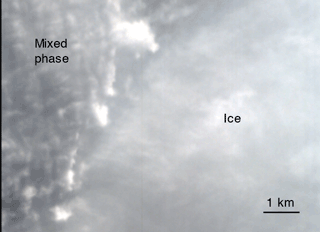Figure 2Typical fragment of a top-of-atmosphere reflectance image, drawn from Hyperion product ID EO1H2221282005350110KF over Ross Island, Antarctica, and displayed in visible red, green, and blue channels.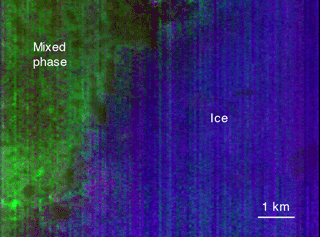Figure 3Thermodynamic phase map corresponding to Fig. 2, with green indicating equivalent liquid thickness and blue indicating equivalent ice thickness.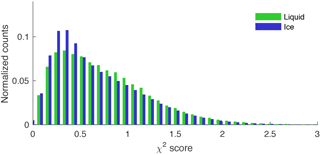Figure 4Normalized histogram of χ2 spectrum fit scores for the entire scene of Figs. 2 and 3. Low χ2 values indicate good fits; χ2=1 is a conservative estimate of measurement noise.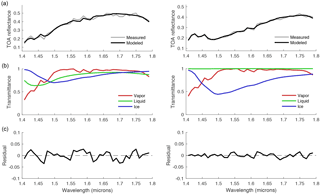Figure 5Spectrum fits from the region of mixed and ice cloud in Fig. 2. (a) Model fit to spectrum. (b) Transmittances for each absorber. (c) Residual error.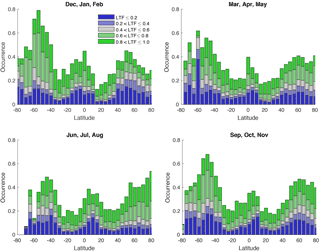Figure 6Zonal average cloud phase, partitioned by season, for all 10 years of observations. Sampling is nonuniform across latitudes; Sect. 3 quantifies uncertainty due to finite sample sizes.

Figure 6 shows the entire distribution of mixed and pure phase clouds for all 10 years of observations and binned in 10 increments, ranging from pure ice (LTF<0.2) to pure liquid (LTF>0.8). Note that Hyperion sampling is nonuniform across histogram bins, and Sect. 3 quantifies uncertainty for different latitudes. The dataset clearly resolved key features apparent in records from other sensors like MODIS and CALIPSO . A band of ice clouds peaked in the Intertropical Convergence Zone at approximately 5–10 latitude, and other seasonally dependent maxima appeared at approximately 60 and 60. The population associated with the LTF range from 0.6 to 0.8 was fairly large and possibly included some pure water pixels that were misclassified due to estimation noise. The mixed phase clouds were most numerous in the middle and extreme high latitudes and less abundant in the tropics. There was a general increase in the occurrence of liquid clouds at middle and high latitudes, with a strong asymmetry near the solstice: a seasonal peak in liquid cloud coverage for the summer months was apparent in both hemispheres.

3 AIRS comparison

## 3.1 Method

Next, we compared the resulting thermodynamic phase retrievals to a decadal dataset of cloud phase retrievals by the AIRS instrument . This was a dramatically different measurement obtained from thermal infrared spectra with a coarse 13.5 km footprint rather than reflected solar energy at fine spatial resolution. detail the algorithm, and validate it using pixel-scale comparisons with CALIPSO data. We filtered clouds using an AIRS sensitivity threshold (effective cloud fraction, or ECF) of 0.1 and binned AIRS phases by latitude and season for direct comparison.

We anticipated several differences in the result. First, AIRS sampled uniformly over the Earth's surface while Hyperion imaged only during the day and favored land areas. We also expected differences in sensitivity; AIRS was far more sensitive to optically thin clouds, while the Hyperion analysis intentionally excluded thin clouds with a strict detection threshold. To help account for these differences, we normalized the relative cloud abundances of both instruments. We designated a reference latitude band from 60 to 60 (the area of densest Hyperion coverage) to have a mean occurrence of unity and compared zonal changes relative to this standard.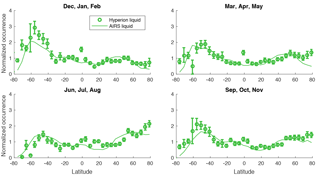Figure 7Comparison of Hyperion and AIRS cloud statistics, both normalized by the 60 to 60 latitude range to account for differences in cloud mask cutoff thresholds. The 10-year seasonal observations are shown separately for the liquid phase. Here Hyperion uses a hard classification; i.e., liquid thickness fractions less than 0.5 are considered ice clouds. Error bars show 95 % confidence intervals calculated via nonparametric bootstrap estimation. The corresponding AIRS error bars would be far smaller due to the large number of samples, so we omit them for clarity.

Additionally, the AIRS algorithm classified ambiguous clouds as “unknown”. This population likely contained mixed phase clouds but also a large fraction of supercooled liquid clouds due to the current AIRS phase algorithm. The liquid tests are based on warm liquid water indices of refraction rather than the supercooled liquid indices of refraction . Following from results of , we reassigned some of the unknown clouds to form 10 % of total ice and 60 % of total liquid. For liquid clouds, we defined a corrected occurrence L in each latitude bin and took just 40 % of this to be from the original AIRS estimate L:

$\begin{array}{}\text{(10)}& \left(\mathrm{1}-\mathrm{0.6}\right)\sum {L}^{\prime }=\sum L,\end{array}$

where summations ran over all latitude bins. This allowed a unique correction for each latitude bin, given as the product of its unknown cloud fraction U and a global multiplicative coefficient α:

$\begin{array}{}\text{(11)}& {L}^{\prime }=L+\mathit{\alpha }U.\end{array}$

Equations (6) and (7) gave

$\begin{array}{}\text{(12)}& \mathit{\alpha }=\frac{\mathrm{0.6}\sum L}{\left(\mathrm{1}-\mathrm{0.6}\right)\sum U}.\end{array}$

We defined a similar relation for ice cloud with a missing fraction of 0.1 rather than 0.6. Together, the two adjustments (magnitude normalization and reassignment of unknown clouds) accounted for known biases, which permitted a comparison of zonal gradients between the two instruments. While we expected some discrepancies due to differences in instruments and sampling, the comparison provided a useful check between two very different measurement techniques.

## 3.2 Results

Figures 7 and 8 show liquid and ice cloud phase spatial distributions across latitudes, partitioned by season, for all 10 years of observations binned in 10 increments. Error bars show 95 % confidence intervals for the mean. Thin lines indicate decadal averages derived from the AIRS dataset. Distributions from the two instruments generally agreed – particularly for ice, to which AIRS was very sensitive. Tropical ice clouds showed the best agreement, as in prior studies comparing AIRS and CALIPSO . Differences may be related to the much stronger sensitivity to thin ice cloud, coarse spatial resolution and near-global daily sampling, and ambiguity of the unknown category with respect to how many liquid, ice, and mixed phase clouds are contained within that categorization. Another potential contributor to the discrepancy is sensitivity to different altitudes in large or multilayer cloud systems. Multilayered clouds are abundant in tropical regions and midlatitude storm tracks. In cases where, for example, a translucent ice cloud overlays an optically thin liquid cloud, the two instruments would measure different thermodynamic phase. For reference, we place a non-normalized comparison of the two instrument datasets in Appendix A.

4 Spatial scale analysis

## 4.1 Method

We next characterized spatial scaling properties of the thermodynamic phase maps. A variogram estimated the expected squared differences between LTF at any two locations x and x in the map as a function v(d) of the lag distance d between the paired points. We used the classical estimator based on the sample variance at each lag distance:

$\begin{array}{}\text{(13)}& v\left(d\right)=\frac{\mathrm{1}}{\mathrm{2}N\left(d\right)}\sum _{‖x-{x}^{\prime }‖=d}{\left(\mathrm{LTF}\left(x\right)-\mathrm{LTF}\left({x}^{\prime }\right)\right)}^{\mathrm{2}},\end{array}$

where N(d) was the number of such points in the sample. The näive formulation involving calculations of all point pairs would have required over 1010 squared differences per scene, an intractable number. Instead, we calculated the same quantity efficiently in the Fourier domain using the method of , with a spatial mask to ensure that only cloud pixels influenced the calculation.

Table 2Model parameters of tropical and extratropical clouds, for $v=a{d}^{b}+c$, with confidence bounds and R squared coefficients.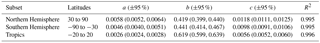We fit the resulting variogram with a power law of the form $v\left(d\right)=a{d}^{b}+c$, subsampling the data to achieve log-constant point density and optimizing free parameters with the Levenberg–Marquardt method. We considered the hypothesis that tropical clouds would have different spatial scaling from extratropical clouds because of the dominant influence of convective versus baroclinic systems. To test this, we analyzed the scenes in three segments: a tropical band within 20 of the Equator and extratropical scenes poleward of 30 north and south latitudes. We used cloud fields larger than a 3 km cutoff, excluding variograms that evaluated to zero at shorter lag distances due to the lack of sufficient cloud pixels in the scene.

## 4.2 Results

Finally, Fig. 9 shows variograms over all years. Each data point corresponds to a lag distance for an aggregate variogram, so separation between the curves does not imply complete separation between populations. However, statistical analysis does reveal some significant differences in scaling properties. Table 2 shows the best-fitting coefficients for variance v in the liquid thickness fraction of tropical clouds, northern hemispheric extratropical clouds, and southern hemispheric extratropical clouds, as a function $v\left(d\right)=a{d}^{b}+c$ of distance d in kilometers. R2 values indicate the power law is an excellent least-squares fit. Parenthesis indicate 95 % confidence intervals on the parameters.

We report scaling exponents in terms of variance; these could be translated to other conventions using structure functions or the power spectrum domain. Differences between the power law exponents for tropical and extratropical clouds were statistically significant. The extratropical scaling exponents of 0.42 and 0.44 are similar to, but slightly in excess of the classic Kolmogorov scaling of 1∕3 ($-\mathrm{5}/\mathrm{3}$ in the power spectral domain). The tropical scaling exponent of 0.62 is in excess of the classic Kolmogorov scaling of 1∕3 but is consistent with tropical cloud reflectance variability reported in and mid-tropospheric water vapor mixing ratio in the tropics from the AIRS instrument, e.g., . At finer spatial resolutions there is also evidence of scale breaks dependent on altitude. Consequently the scaling exponent is dependent on the length scale range calculated .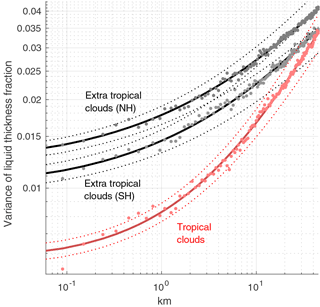Figure 9Variogram for all clouds in the Hyperion dataset, showing the variance as a function of separation between points. The curve suggests a power law relationship. Dotted lines indicate 95 % confidence intervals on the coefficients.

The additive offset c defined the variance at zero distance, i.e., the irreducible measurement error for each spectrum. This implied a noise-equivalent change in liquid water fraction of approximately 7.5 % for tropical and 10–11 % for extratropical clouds. The addend and pre-factor coefficients differed significantly between extratropical and tropical clouds and to a much smaller degree between the two extratropical populations. All three populations were subject to Hyperion's biased sampling of ocean and land and of instrument noise conditions dominated by solar zenith angles. We would not ascribe differences between northern and southern hemispheres to cloud scaling since these discrepancies were small relative to their separation from the tropical model. In all cases, measurement error was a minor contributor to observed variance – most variability arose from spatially correlated structure. Outside the tropics, measurement error accounted for just 25 % of variance observed at GCM grid scales of 100 km. The remaining 75 % was therefore attributable to spatial structure at subgrid scales.

5 Conclusions

This study reports the first global high spatial resolution survey of cloud thermodynamic phase. The Hyperion imaging spectrometer provides two novel contributions beyond prior records. First, highly diagnostic spectral features permit accurate discrimination of mixed phase clouds. Second, horizontal scales down to 30 m capture the characteristic spatial scaling relationships of the thermodynamic phase field. Aggregate seasonal and latitudinal changes of cloud thermodynamic phase generally corroborate observations by other sensing modalities, such as those of AIRS. Variogram analysis reveals a noise-equivalent measurement error of 7.5–11 % in the liquid thickness fraction for different latitudinal zones. Spatial correlations follow a power law relationship with approximately 50 % measurable variance determined at length scales of 6 km. Significant spatial variability appears at scales far below the resolution of typical GCMs.

We note an important caveat to these results. The Hyperion datasets were spatially biased and strongly favored land mass over ocean. Insofar as the latitudinal distributions show asymmetries across northern and southern hemispheres, this may be related to the spatial distribution of land mass in the southern hemispheric midlatitude areas. Southern Hemisphere observations were often acquired over islands, which would exhibit a more oceanic influence on cloud cover.

Despite this qualification, the Hyperion results generally corroborate existing global datasets where there is overlap, a useful complement to instruments measuring cloud phase with polarization or thermal emission. They provide a “first of its kind” observational record at sub-kilometer scales. These scales are critical for advancing subgrid parameterizations in GCMs, including the Wegener–Bergeron–Findeisen timescale of the growth of ice crystals and numerous other temperature-dependent microphysical processes that control ice and liquid water partitioning , and for further extending the observational record to a new extreme of spatial resolution.

Data availability

Appendix A: Non-normalized AIRS comparisons

Figures A1 and A2 show AIRS zonal averages for liquid and ice, respectively, with the correction of but without normalization across latitudes. Thin lines indicate AIRS frequencies for ECF thresholds of 0.1 and 0.5. This indicates the sensitivity of the AIRS result to this choice. The absolute abundance of the ice phase shows the largest disparities. This is expected and accountable to very thin ice clouds to which AIRS is far more sensitive.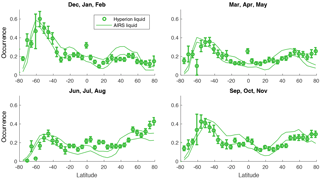Figure A1A comparison similar to Fig. 7 without normalization across latitudes. Two thin lines represent AIRS retrievals using the standard effective cloud fraction retrieval threshold of 0.5 and 0.1.

Competing interests

The authors declare that they have no conflict of interest.

Acknowledgements

This research was performed at the Jet Propulsion Laboratory, California Institute of Technology, under a contract with the National Aeronautics and Space Administration. We thank the Hyperion Team of Goddard Space Flight Center for their assistance in acquiring and interpreting the data. We thank Bo-Cai Gao for radiative transfer methods used in part of the Hyperion analysis. US Government support acknowledged.

Edited by: Alexander Kokhanovsky
Reviewed by: four anonymous referees

References

Barker, H. W., Qu, Z., Bélair, S., Leroyer, S., Milbrandt, J. A., and Vaillancourt, P. A.: Scaling properties of observed and simulated satellite visible radiances, J. Geophys. Res.-Atmos., 122, 9413–9429, 2017. a

Bender, F. A., Ramanathan, V., and Tselioudis, G.: Changes in extratropical storm track cloudiness 1983–2008: observational support for a poleward shift, Clim. Dynam., 38, 2037–2053, 2012. a

Boardman, J. W. and Kruse, F. A.: Analysis of Imaging Spectrometer Data Using N-Dimensional Geometry and a Mixture-Tuned Matched Filtering Approach, IEEE T. Geosci. Remote, 49, 4138–4152, 2011. a

Brown, L. D. and Levine, M.: Variance estimation in nonparametric regression via the difference sequence method, Ann. Stat., 35, 2219–2232, 2007. a

Ceppi, P. and Hartmann, D. L.: Connections between clouds, radiation, and midlatitude dynamics: A review, Current Climate Change Reports, 1, 94–102, 2015. a

Ceppi, P., Hartmann, D. L., and Webb, M. J.: Mechanisms of the negative shortwave cloud feedback in middle to high latitudes, J. Climate, 29, 139–157, 2016. a

Chylek, P., Robinson, S., Dubey, M., King, M., Fu, Q., and Clodius, W.: Comparison of near-infrared and thermal infrared cloud phase detections, J. Geophys. Res., 111, D20203, https://doi.org/10.1029/2006JD007140, 2006. a

Dudhia, A.: Oxford University Reference Forward Model (RFM), available at: http://www.atm.ox.ac.uk/RFM/ (last access: 15 February 2018), 2014. a

Ehrlich, A., Bierwirth, E., Wendisch, M., Gayet, J.-F., Mioche, G., Lampert, A., and Heintzenberg, J.: Cloud phase identification of Arctic boundary-layer clouds from airborne spectral reflection measurements: test of three approaches, Atmos. Chem. Phys., 8, 7493–7505, https://doi.org/10.5194/acp-8-7493-2008, 2008. a

Eldering, A., O'Dell, C. W., Wennberg, P. O., Crisp, D., Gunson, M. R., Viatte, C., Avis, C., Braverman, A., Castano, R., Chang, A., Chapsky, L., Cheng, C., Connor, B., Dang, L., Doran, G., Fisher, B., Frankenberg, C., Fu, D., Granat, R., Hobbs, J., Lee, R. A. M., Mandrake, L., McDuffie, J., Miller, C. E., Myers, V., Natraj, V., O'Brien, D., Osterman, G. B., Oyafuso, F., Payne, V. H., Pollock, H. R., Polonsky, I., Roehl, C. M., Rosenberg, R., Schwandner, F., Smyth, M., Tang, V., Taylor, T. E., To, C., Wunch, D., and Yoshimizu, J.: The Orbiting Carbon Observatory-2: first 18 months of science data products, Atmos. Meas. Tech., 10, 549–563, https://doi.org/10.5194/amt-10-549-2017, 2017. a

Gao, B.-C. and Goetz, A. F.: Retrieval of equivalent water thickness and information related to biochemical components of vegetation canopies from AVIRIS data, Remote Sens. Environ., 52, 155–162, 1995. a

Garrigues, S., Allard, D., Baret, F., and Weiss, M.: Quantifying spatial heterogeneity at the landscape scale using variogram models, Remote Sens. Environ., 103, 81–96, 2006. a

Griffin, M. K., hua K. Burke, H., Mandl, D., and Miller, J.: Cloud Cover Detection Algorithm for EO-1 Hyperion Imagery, Algorithms and Technologies for Multispectral, Hyperspectral, and Ultraspectral Imagery IX, IEEE International Geoscience and Remote Sensing Symposium, 21–25 July 2003, Toulouse, France, Proceedings, 1, 86–89, https://doi.org/10.1109/IGARSS.2003.1293687, 2003. a, b

Hirakata, M., Okamoto, H., Hagihara, Y., Hayasaka, T., and Oki, R.: Comparison of global and seasonal characteristics of cloud phase and horizontal ice plates derived from CALIPSO with MODIS and ECMWF, J. Atmos. Ocean. Tech., 31, 2114–2130, 2014. a

Hu, Y., Rodier, S., Xu, K.-M., Sun, W., Huang, J., Lin, B., Zhai, P., and Josset, D.: Occurrence, liquid water content, and fraction of supercooled water clouds from combined CALIOP/IIR/MODIS measurements, J. Geophys. Res., 115, D00H34, https://doi.org/10.1029/2009JD012384, 2010. a

Jin, H. and Nasiri, S. L.: Evaluation of AIRS Cloud-Thermodynamic-Phase Determination with CALIPSO, J. Appl. Meteorol. Clim., 53, 1012–2027, 2014. a, b, c, d

Kahn, B. H., Teixeira, J., Fetzer, E., Gettelman, A., Hristova-Veleva, S., Huang, X., Kochanski, A., Köhler, M., Krueger, S., Wood, R., and Zhao, M.: Temperature and water vapor variance scaling in global models: Comparisons to satellite and aircraft data, J. Atmos. Sci., 68, 2156–2168, 2011. a, b

Kahn, B. H., Irion, F. W., Dang, V. T., Manning, E. M., Nasiri, S. L., Naud, C. M., Blaisdell, J. M., Schreier, M. M., Yue, Q., Bowman, K. W., Fetzer, E. J., Hulley, G. C., Liou, K. N., Lubin, D., Ou, S. C., Susskind, J., Takano, Y., Tian, B., and Worden, J. R.: The Atmospheric Infrared Sounder version 6 cloud products, Atmos. Chem. Phys., 14, 399–426, https://doi.org/10.5194/acp-14-399-2014, 2014. a, b

Kokhanovsky, A.: Optical properties of terrestrial clouds, Earth-Sci. Rev., 64, 189–241, 2004. a

Kou, L., Labrie, D., and Chylek, P.: Refractive indices of water and ice in the 0.65-to 2.5-µm spectral range, Appl. Optics, 32, 3531–3540, 1993. a

Lawson, C. L. and Hanson, R. J.: Solving least squares problems, Prentice-Hall, Inc., Englewood Cliffs, New Jersey, USA, 337 pp., 1974. a

Marcotte, D.: Fast Variogram Computation with FFT, Comput. Geosci., 22, 1175–1186, 1996. a

Martins, J. V., Marshak, A., Remer, L. A., Rosenfeld, D., Kaufman, Y. J., Fernandez-Borda, R., Koren, I., Correia, A. L., Zubko, V., and Artaxo, P.: Remote sensing the vertical profile of cloud droplet effective radius, thermodynamic phase, and temperature, Atmos. Chem. Phys., 11, 9485–9501, https://doi.org/10.5194/acp-11-9485-2011, 2011. a

Marvel, K., Zelinka, M., Klein, S. A., Bonfils, C., Caldwell, P., Doutriaux, C., Santer, B. D., and Taylor, K. E.: External influences on modeled and observed cloud trends, J. Climate, 28, 4820–4840, 2015. a

McCoy, D. T., Hartmann, D. L., Zelinka, M. D., Ceppi, P., and Grosvenor, D. P.: Mixed-phase cloud physics and Southern Ocean cloud feedback in climate models, J. Geophys. Res.-Atmos., 120, 9539–9554, 2015. a

Mouroulis, P., Green, R. O., Van Gorp, B., Moore, L. B., Wilson, D. W., and Bender, H. A.: Landsat swath imaging spectrometer design, Opt. Eng., 55, 015104, https://doi.org/10.1117/1.OE.55.1.015104, 2016. a

National Aeronautics and Space Administration: EO-1 Hyperion Level 1 Dataset, available at: http://earthexplorer.usgs.gov, last access: 15 February 2018. a

Norris, J. R., Allen, R. J., Evan, A. T., Zelinka, M. D., O'Dell, C. W., and Klein, S. A.: Evidence for climate change in the satellite cloud record, Nature, 536, 72–75, 2016. a

Pagano, T. S., Aumann, H. H., Hagan, D. E., and Overoye, K.: Prelaunch and in-flight radiometric calibration of the Atmospheric Infrared Sounder (AIRS), IEEE T. Geosci. Remote, 41, 265–273, 2003. a

Pilewskie, P. and Twomey, S.: Cloud phase discrimination by reflectance measurements near 1.6 and 2.2 µm, J. Atmos. Sci., 44, 3419–3420, 1987. a

Rothman, L., Gordon, I., Babikov, Y., Barbe, A., Chris Benner, D., Bernath, P., Birk, M., Bizzocchi, L., Boudon, V., Brown, L., Campargue, A., Chance, K., Cohen, E. A., Coudert, L. H., Devi, V. M., Drouin, B. J., Fayt, A., Flaud, J.-M., Gamache, R. R., Harrison, J. J., Hartmann, J.-M., Hill, C., Hodges,J. T., Jacquemart, D., Jolly, A., Lamouroux, J., Le Roy, R. J., Li, G., Long, D. A., Lyulin, O. M., Mackie, C. J., Massie, S. T., Mikhailenko, S., Müller, H. S. P., Naumenko, O. V., Nikitin, A. V., Orphal, J., Perevalov, V., Perrin, A., Polovtseva, E. R., Richard, C., Smith, M. A. H., Starikova, E., Sung, K., Tashkun, S., Tennyson, J., Toon, G. C., Tyuterev, V. G., and Wagner, G.: The HITRAN2012 molecular spectroscopic database, J. Quant. Spectros. Ra., 130, 4–50, 2013.  a

Rowe, P. M., Neshyba, S., and Walden, V. P.: Radiative consequences of low-temperature infrared refractive indices for supercooled water clouds, Atmos. Chem. Phys., 13, 11925–11933, https://doi.org/10.5194/acp-13-11925-2013, 2013. a

Tan, I. and Storelvmo, T.: Sensitivity Study on the Influence of Cloud Microphysical Parameters on Mixed-Phase Cloud Thermodynamic Phase Partitioning in CAM5, J. Atmos. Sci., 73, 709–727, 2016. a, b

Tan, I., Storelvmo, T., and Zelinka, M. D.: Observational constraints on mixed-phase clouds imply higher climate sensitivity, Science, 352, 224–227, 2016. a

Thompson, D. R., Gao, B.-C., Green, R. O., Roberts, D. A., Dennison, P. E., and Lundeen, S.: Atmospheric correction for global mapping spectroscopy: ATREM advances for the HyspIRI preparatory campaign, Remote Sens. Environ., 167, 64–77, https://doi.org/10.1016/j.rse.2015.02.010, 2015. a, b

Thompson, D. R., McCubbin, I., Gao., B.-C., Green, R. O., Matthews, A. A., Mei, F., Meyer, K., Platnick, S., Schmid, B., Tomlinson, J., and Wilcox, E.: Measuring cloud thermodynamic phase with shortwave infrared imaging spectroscopy, J. Geophys. Res.-Atmos., 121, 9174–9190, https://doi.org/10.1002/2016JD024999, 2016. a, b, c, d, e, f, g

Von Neumann, J.: Distribution of the ratio of the mean square successive difference to the variance, Ann. Math. Stat., 12, 367–395, 1941. a

Wasserman, L.: All of Nonparametric Statistics, Springer-Verlag, New York, USA, 2006. a